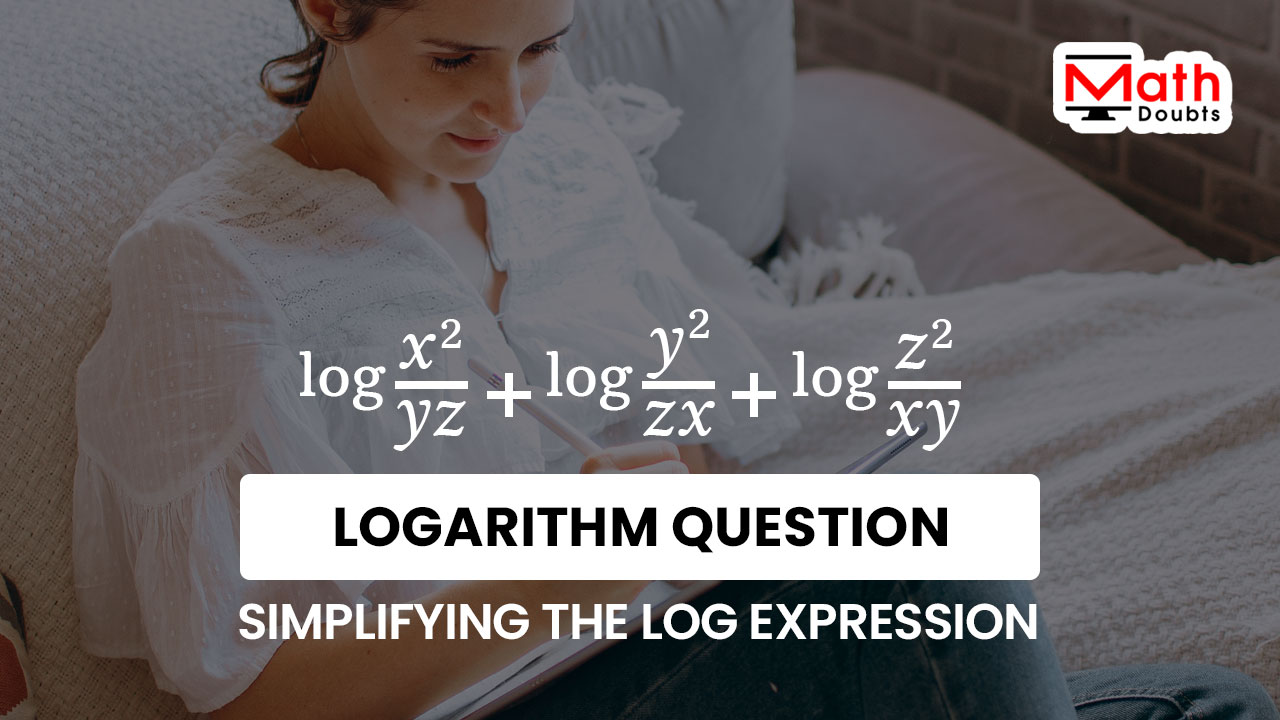# Evaluate $\log{\dfrac{x^2}{yz}}$ $+$ $\log{\dfrac{y^2}{zx}}$ $+$ $\log{\dfrac{z^2}{xy}}$The common logarithm of $x$ square divided by the product of $y$ and $z$, plus the common logarithm of the quotient of $y$ square divided by $z$ times $x$, plus common logarithm of square of $z$ divided by the product of $x$ and $y$ is the given logarithmic expression in algebraic form and it should be evaluated by simplifying it in this logarithmic question.

### Expand the Logarithm of the Quotients

The quantities in the common logarithm are in rational form in all three terms. The logarithm of a quotient can be expanded as the difference of their logarithms as per the quotient rule of the logarithms in the logarithmic expression in algebraic form.

$=\,\,$ $\log{x^2}$ $-$ $\log{(yz)}$ $+$ $\log{y^2}$ $-$ $\log{(zx)}$ $+$ $\log{z^2}$ $-$ $\log{(xy)}$

### Remove the exponents from power functions

There are power functions inside the common logarithm in first, third and fifth terms of the logarithmic expression. Now, use the power rule of the logarithms to eliminate the exponents from the power functions inside the common logarithm.

$=\,\,$ $2 \times \log{x}$ $-$ $\log{(yz)}$ $+$ $2 \times \log{y}$ $-$ $\log{(zx)}$ $+$ $2 \times \log{z}$ $-$ $\log{(xy)}$

$=\,\,$ $2\log{x}$ $-$ $\log{(yz)}$ $+$ $2\log{y}$ $-$ $\log{(zx)}$ $+$ $2\log{z}$ $-$ $\log{(xy)}$

### Expand the Logarithm of the Products

Two quantities in algebraic form are multiplying inside the logarithm in second, fourth and sixth terms of the logarithmic expression in algebraic form. The logarithm of a product of two quantities can be expanded as sum of their logs, as per the product rule of the logarithms.

$=\,\,$ $2\log{x}$ $-$ $(\log{y}$ $+$ $\log{z})$ $+$ $2\log{y}$ $-$ $(\log{z}$ $+$ $\log{x})$ $+$ $2\log{z}$ $-$ $(\log{x}$ $+$ $\log{y})$

### Evaluate the Log expression by simplification

Finally, it is time to simplify the logarithmic expression in algebraic form for finding the value of the given logarithmic expression.

$=\,\,$ $2\log{x}$ $-$ $\log{y}$ $-$ $\log{z}$ $+$ $2\log{y}$ $-$ $\log{z}$ $-$ $\log{x}$ $+$ $2\log{z}$ $-$ $\log{x}$ $-$ $\log{y}$

According to the commutative property, the places of the terms within the expression can be changed and it helps us to write the like logarithmic terms closer.

$=\,\,$ $2\log{x}$ $-$ $\log{x}$ $-$ $\log{x}$ $+$ $2\log{y}$ $-$ $\log{y}$ $-$ $\log{y}$ $+$ $2\log{z}$ $-$ $\log{z}$ $-$ $\log{z}$

The like logarithmic terms can be added by the addition rule of the like terms and it simplifies the logarithmic expression.

$=\,\,$ $2\log{x}$ $-$ $2\log{x}$ $+$ $2\log{y}$ $-$ $2\log{y}$ $+$ $2\log{z}$ $-$ $2\log{z}$

Now, let us write the like logarithmic terms as groups to simplify the logarithmic expression further.

$=\,\,$ $(2\log{x}$ $-$ $2\log{x})$ $+$ $(2\log{y}$ $-$ $2\log{y})$ $+$ $(2\log{z}$ $-$ $2\log{z})$

$=\,\,$ $(\cancel{2\log{x}}$ $-$ $\cancel{2\log{x}})$ $+$ $(\cancel{2\log{y}}$ $-$ $\cancel{2\log{y}})$ $+$ $(\cancel{2\log{z}}$ $-$ $\cancel{2\log{z}})$

$=\,\,$ $0+0+0$

$=\,\,$ $0$

Latest Math Topics
Jun 26, 2023
Jun 23, 2023

###### Math Questions

The math problems with solutions to learn how to solve a problem.

Learn solutions

Practice now

###### Math Videos

The math videos tutorials with visual graphics to learn every concept.

Watch now

###### Subscribe us

Get the latest math updates from the Math Doubts by subscribing us.